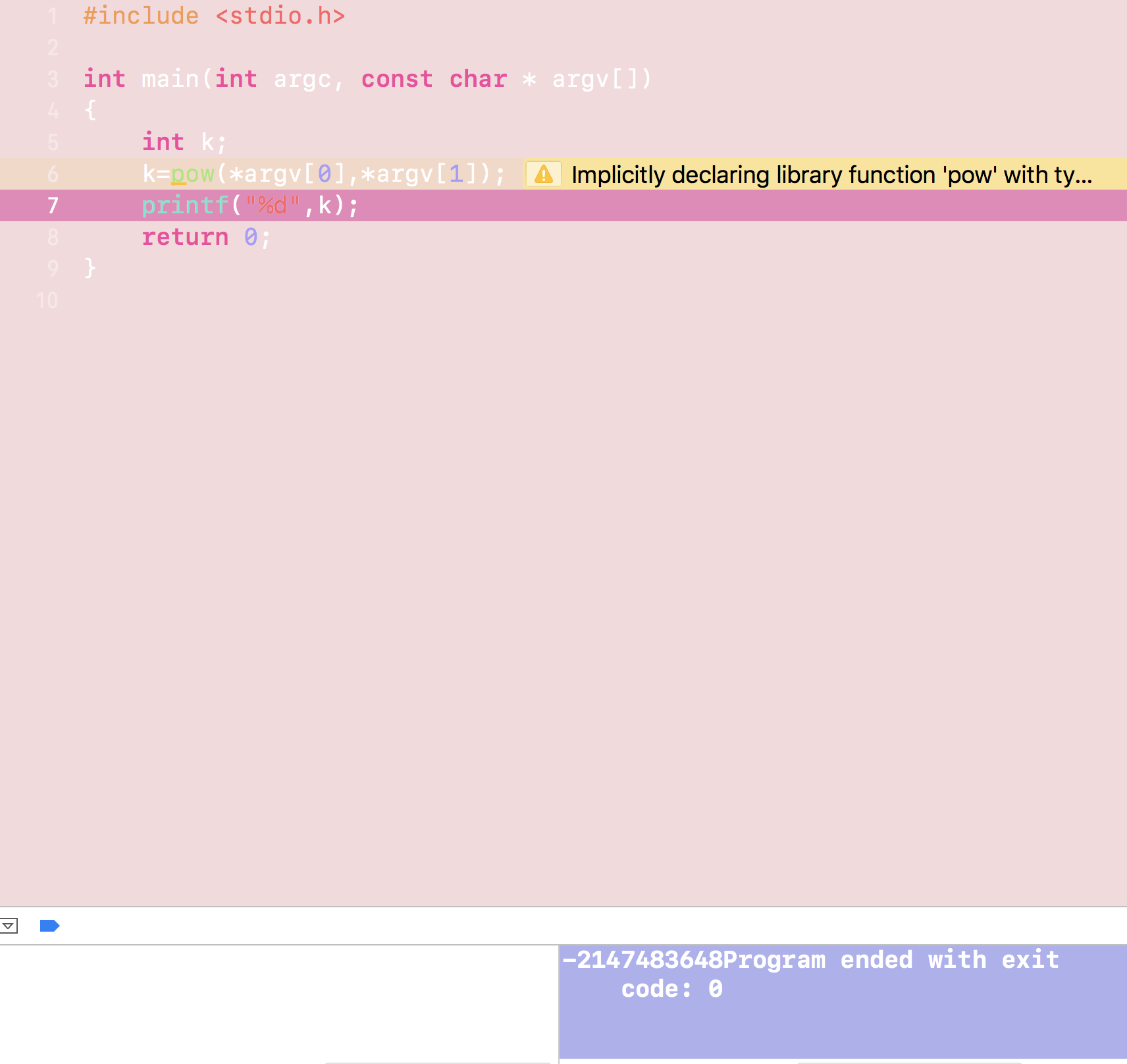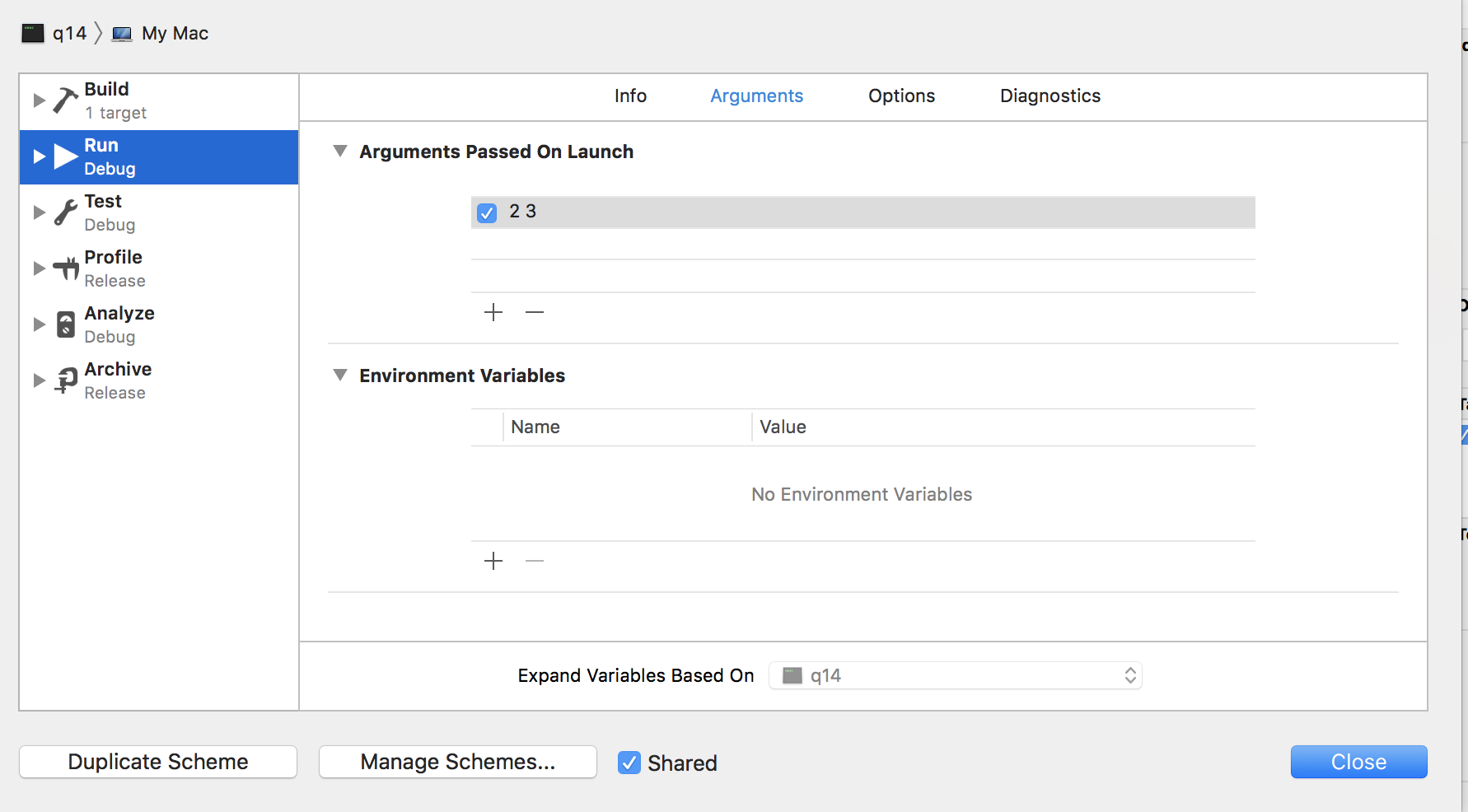2019-04-03 01:37

# c编写一个通过命令行运行的程序计算幂,可是不论参数输入什么，都是输出的这个结果，请问我哪里写错了？

40• 点赞
• 写回答
• 关注问题
• 收藏
• 复制链接分享
• 邀请回答

#### 3条回答

•回答这么多问题就耍赖把我的积分一笔勾销了 2年前
``````你选的字体颜色对比度太低！下次请贴出文字格式的代码（用"代码片"）工具格式化下

你的输入的参数是字符串类型
你需要用atoi或者atof转换成浮点数

pow的结果是浮点数，输出的时候用 %f，而不是%d

:#include <math.h>
#include <stdio.h>
#include <stdlib.h>
int main(int argc, const char * argv[])
{
float a = atof(argv);
float b = atof(argv);
float k = pow(a, b);
printf("%f", k);
return 0;
}
``````
点赞 1 评论 复制链接分享
• 楼上有道理，
但argv是程序本身，
应改成argv和argv:

``````#include <math.h>
#include <stdio.h>
#include <stdlib.h>
int main(int argc, const char * argv[])
{
float a = atof(argv);
float b = atof(argv);
float k = pow(a, b);
printf("%f", k);
return 0;
}
``````
点赞 评论 复制链接分享
• 提示了没有pow这个函数声明，如果使用需要添加对应头文件。

``````#include<math.h>
``````
点赞 评论 复制链接分享